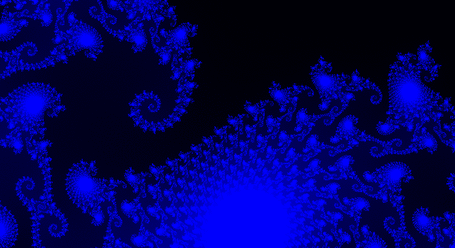A# Julia setJulia set from (-.1, -.5) to (1, .1). Image credit: Andrew Cantino.

A Julia set is any of any infinite number of fractal sets of points on the complex plane (see Argand diagram) defined by a simple rule. Given two complex numbers z and c, and the recursion zn+1 = zn2 + c, the Julia set for any given value of c, consists of all values of z for which z, when iterated in the equation above, does not "blow up" or tend to infinity. Julia sets are closely related to the Mandelbrot set, which is the set of all values of c for which z = 0 + 0i doesn't tend to infinity through application of the recursion. The Mandelbrot set is, in a way, an index of all Julia sets, For any point on the complex plane (which represents a value of c) a corresponding Julia set can be drawn. We can imagine a movie of a point moving about the complex plane with its corresponding Julia set. When the point lies inside the Mandelbrot set the corresponding Julia set is topologically unified or connected. As the point crosses the boundary of the Mandelbrot set, the Julia set explodes into a cloud of disconnected points called Fatou dust. If c is on the boundary of the Mandelbrot set, but not a waist point, the Julia set of c looks like the Mandelbrot set in sufficiently small neighborhoods of c.

Julia sets are named after the French mathematician Gaston Julia (1893–1978), whose most famous work, Memoire sur l'iteration des fonctions rationnelles, which provides the theory for Julia sets before computers were available to computer or represent them, was written in a hospital in 1918 at the age of twenty-five. As a soldier in World War I Julia had been severely wounded and lost his nose. He wrote his greatest treatise between the painful operations necessitated by his wounds.